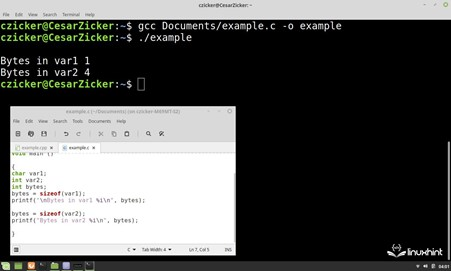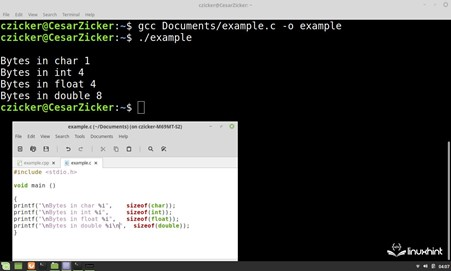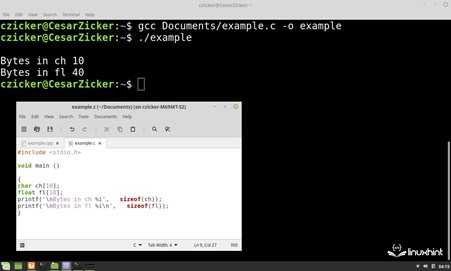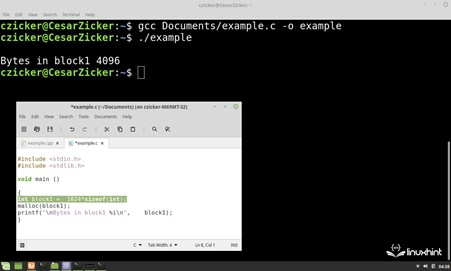| by Arround The Web | No comments

## Sizeof() Operator in C Language

In C language, the data type that is assigned to a variable is directly related to the size in bytes that it occupies in the memory. For example, the data type char or unsigned char always occupies 1 byte, but the other primitive data types such as an integer of type int occupy 4 bytes on a 32-bit system while they occupy 8 bytes on a 64-bit system.

The size of variables and arrays in bytes depends on the type of data and the system on which the program is running.

This factor is important because the variables, matrices, structures, or blocks that are used by the various functions in the C language such as the dynamic memory management, files, sockets, and many others manage the volume of their buffers in bytes. Therefore, to be able to use them, it’s necessary to obtain this value through an operation that calculates the size of the buffer.

In this Linuxhint article, you’ll learn how to use the unary sizeof() operator to determine the size in bytes of a variable, array, or data structure. You’ll see a theoretical description of the syntax and how this operator works. Then, we’ll apply what we learned in practical examples with code snippets and images where we’ll show you the most common uses of this operator in different cases.

Syntax of the Sizeof() Operator in C Language

sizeof (operator)

## Description of the Sizeof() Operator in C Language

The sizeof() operator returns a size_t which contains the number of bytes that is occupied by a particular data type, variable, array, structure, or memory block. In each case, the return value is the result of the sizeof() operator which is applied to one of these.

Next, let’s look at some examples that show how to use this operator to determine the size of various objects.

## How to Determine the Size of a Variable in Bytes with the Sizeof() Operator

How do we determine the size of a variable in bytes with the sizeof() operator in C language?

The size of a variable in bytes is obtained as the result of the sizeof() operation on the variable that is specified by its identifier in the operand. The following code shows how this operator works with char and int. The result of each operation is stored in the variable bytes and then displayed on the command console using the printf() function.

#include <stdio.h>

void main ()

{
char var1;
int var2;
int bytes;
bytes = sizeof(var1);
printf("Bytes in var1 %i", bytes);

bytes = sizeof(var2);
printf("Bytes in var2 %i", bytes);

}

The following image shows the compilation and execution of this code where you can see the result which corresponds to the number of bytes that each variable occupies according to its data type:## How to Use the Sizeof() Operator in the C Language to Determine the Size of a Given Data Type in Bytes

The size of a given data type in bytes is obtained when the sizeof() operator is applied to the type declaration. For example, the following operation obtains the size of a data type int:

sizeof (int)

In the following code, the printf() function displays the result of the sizeof() operator which operates on several data types:

#include <stdio.h>

void main ()

{
printf("\nBytes in char %i",      sizeof(char));
printf("\nBytes in int %i",       sizeof(int));
printf("\nBytes in float %i",     sizeof(float));
printf("\nBytes in double %i\n",  sizeof(double));
}

The following image shows the result of compiling and executing this code where you can see the size that is returned by the sizeof() operator in bytes for each data type:## How to Use the Sizeof() Operator to Get the Size of Arrays with Several Data Types

The sizeof() operator can also determine the size in bytes that an array of elements occupies. In this case, the operand of sizeof() is the identifier of the array whose size in bytes is to be determined. The following code determines and outputs the number of bytes that is occupied by the “ch” array with 10 elements of type char and the “fl” array with the same number of elements of type float:

#include <stdio.h>

void main ()

{
char ch;
float fl;
printf("\nBytes in ch %i",   sizeof(ch));
printf("\nBytes in fl %i\n",   sizeof(fl));
}

The following image shows the compilation and execution of this code. As we can see, the “ch” array occupies 10 bytes while the “fl” array occupies 40:## How to Manage the Dynamic Memory Based on Data Type with the Sizeof() Operator

Dynamic memory management consists of reserving and using the managed memory blocks at runtime.

When reserving and managing a block, we must consider what kind of data we want to store in it, since the size of the block depends directly on it. The number of bytes that are needed for a block of a given data type is obtained by multiplying the number of elements that we want to reserve by the result of the sizeof() which is returned for the type of data that we want to store.

In the following code, the malloc() function reserves 1024 data of type int in block1. The size of the block in bytes is 1024 x sizeof(int):

#include <stdlib.h>
#include <stdio.h>

void main ()

{
int block1 =  1024*sizeof(int);
malloc( 1024*sizeof(int))
printf("\nBytes in block1 %i\n",  block1);
}

The following image shows the compilation and execution of this code which allocates a memory block of 1024 integers and then displays the number of bytes that is occupied by the reserved portion:## Conclusion

In this Linuxhint article, we showed you how to use the unary sizeof() operator to determine the size of an object. We explained its operation and syntax in a theoretical section. We also showed you how important it is to know the byte size of objects and arrays in order to use the different functions in this language.

Also, we showed you with practical examples with code snippets and pictures on how to use the operator in the most common cases where we use the different types of operands and call the different functions where the parameter that is determined with this operator is used as input argument.

Source: linuxhint.com